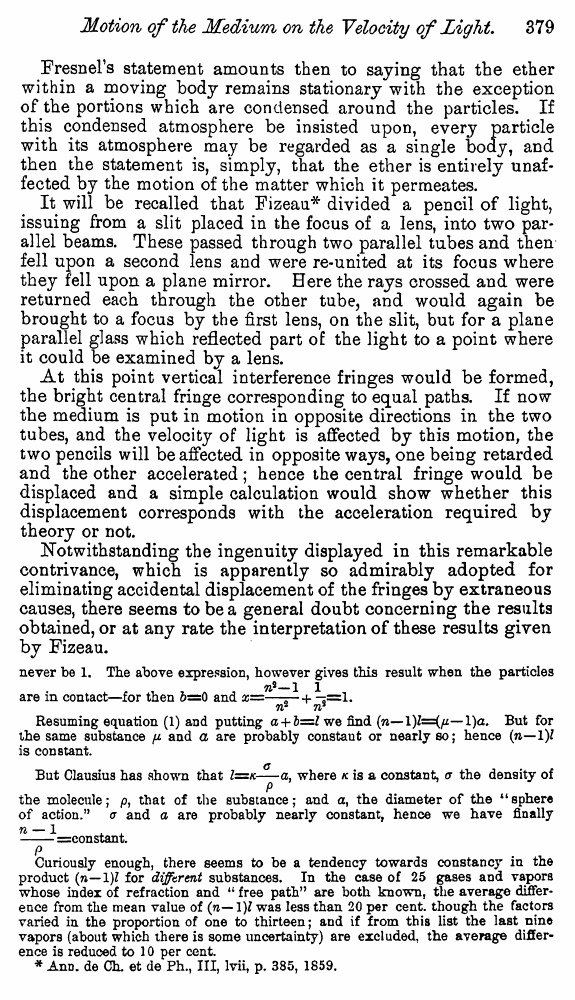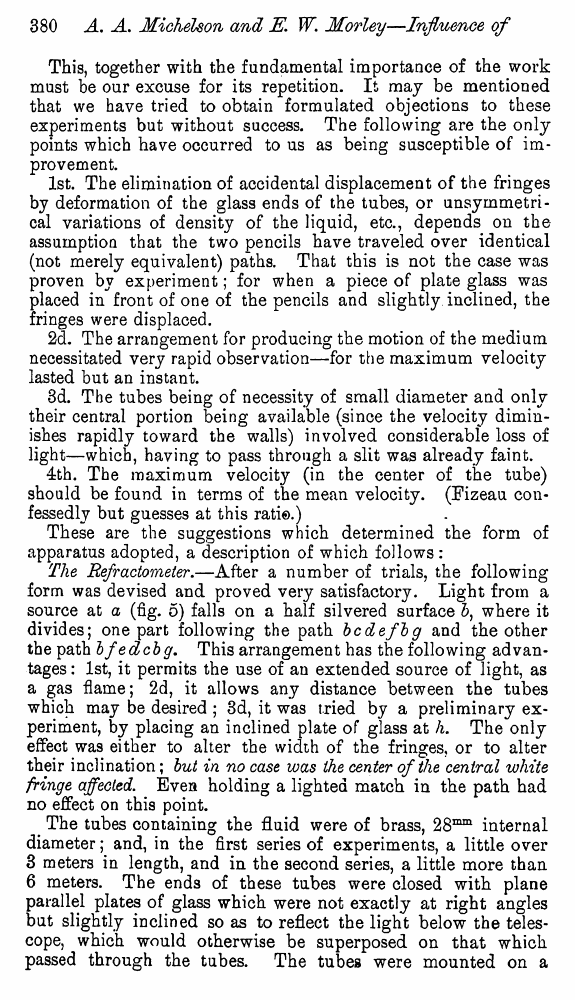# Michelson A.A., Morley E. Influence of Motion of the Medium on the velocity of Light. // American Journal of Science, Ser. 3, 1886, vol. 31

В начало   Другие форматы   <<<     Страница 379   >>>377  378  379 380  381  382  383  384  385  386 Fresnel’s statement amounts then to saying that the ether within a moving body remains stationary with the exception of the portions which are condensed around the particles. If this condensed atmosphere be insisted upon, every particle with its atmosphere may be regarded as a single body, and then the statement is, simply, that the ether is entirely unaffected by the motion of the matter which it permeates. It will be recalled that Fizeau* divided a pencil of light, issuing from a slit placed in the focus of a lens, into two parallel beams. These passed through two parallel tubes and then fell upon a second lens and were re-united at its focus where they fell upon a plane mirror. Here the rays crossed and were returned each through the other tube, and would again be brought to a focus by the first lens, on the slit, but for a plane parallel glass which reflected part o£ the light to a point where it could be examined by a lens. At this point vertical interference fringes would be formed, the bright central fringe corresponding to equal paths. If now the medium is put in motion in opposite directions in the two tubes, and the velocity of light is affected by this motion, the two pencils will be affected in opposite ways, one being retarded and the other accelerated; hence the central fringe would be displaced and a simple calculation would show whether this displacement corresponds with the acceleration required by theory or not. Notwithstanding the ingenuity displayed in this remarkable contrivance, which is apparently so admirably adopted for eliminating accidental displacement of the fringes by extraneous causes, there seems to be a general doubt concerning the results obtained, or at any rate the interpretation of these results given by Fizeau. never be 1. The above expression, however gives this result when the particles 7l2—1 1 are in contact—for then 5=0 and x=—— + -*=1. 71 71 Resuming equation (1) and putting a + b=l we find (w— l)a. But for the same substance p and a are probably constant or nearly so; hence (n—1)1 is constant. But Clausius has shown that Z=/c-^-a, where k is a constant, a the density of 9 the molecule; p, that of the substance; and a, the diameter of the “sphere of action.” a and a are probably nearly constant, hence we have finally n — 1 . . -=constant. P . ^ Curiously enough, there seems to be a tendency towards constancy in the product (ti—1)? for different substances. In the case of 25 gases and vapors whose index of refraction and “ free path” are both known, the average difference from the mean value of (n— 1)J was less than 20 per cent, though the factors varied in the proportion of one to thirteen; and if from this list the last nine vapors (about which there is some uncertainty) are excluded, the average difference is reduced to 10 per cent. * AnD. de Ch. et de Ph., Ill, lvii, p. 385, 1S59. This, together with the fundamental importance of the work must be our excuse for its repetition. It may be mentioned that we have tried to obtain formulated objections to these experiments but without success. The following are the only points which have occurred to us as being susceptible of improvement. 1st. The elimination of accidental displacement of the fringes by deformation of the glass ends of the tubes, or unsymmetri-cal variations of density of the liquid, etc., depends on the assumption that the two pencils have traveled over identical (not merely equivalent) paths. That this is not the case was proven by experiment; for when a piece of plate glass was placed in front of one of the pencils and slightly inclined, the fringes were displaced. 2d. The arrangement for producing the motion of the medium necessitated very rapid observation—for the maximum velocity lasted but an instant. 3d. The tubes being of necessity of small diameter and only their central portion being available (since the velocity diminishes rapidly toward the walls) involved considerable loss of light—which, having to pass through a slit was already faint. 4th. The maximum velocity (in the center of the tube) should be found in terms of the mean velocity. (Fizeau confessedly but guesses at this rati©.) These are the suggestions which determined the form of apparatus adopted, a description of which follows : The Refractometer.—After a number of trials, the following form was devised and proved very satisfactory. Light from a source at a (fig. 5) falls on a half silvered surface 5, where it divides; one part following the path bcdefbg and the other the path bfedcbg. This arrangement has the following advantages : 1st, it permits the use of an extended source of light, as a gas flame; 2d, it allows any distance between the tubes which may be desired ; 3d, it was tried by a preliminary experiment, by placing an inclined plate of glass at h. The only effect wa9 either to alter the width of the fringes, or to alter their inclination; but in no case was the center of the central white fringe affected. Even holding a lighted match in the path had no effect on this point. The tubes containing the fluid were of brass, 28mm internal diameter; and, in the first series of experiments, a little over 3 meters in length, and in the second series, a little more than 6 meters. The ends of these tubes were closed with plane parallel plates of glass which were not exactly at right angles but slightly inclined so as to reflect the light below the telescope, which would otherwise be superposed on that which passed through the tubes. The tubes were mounted on a